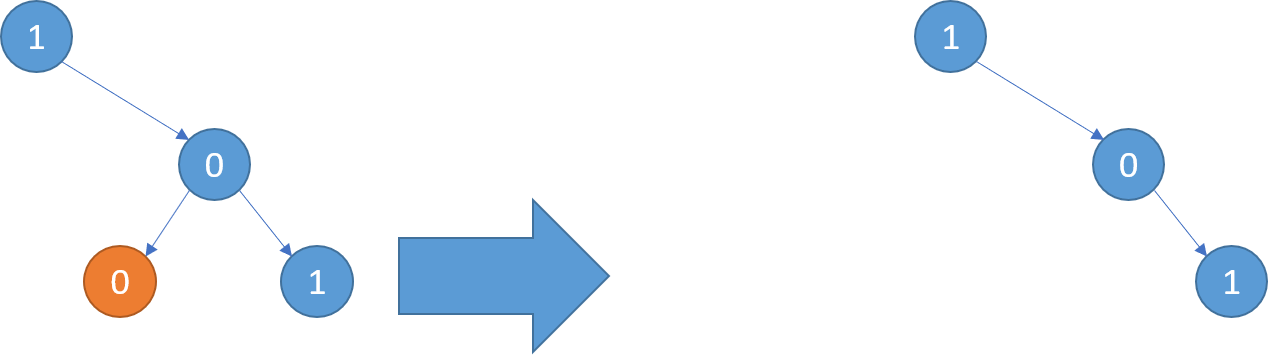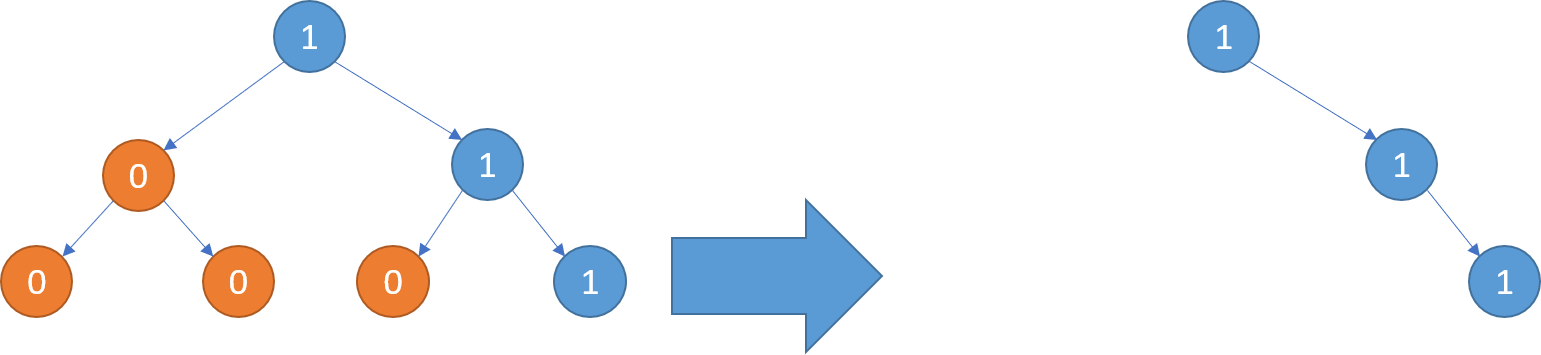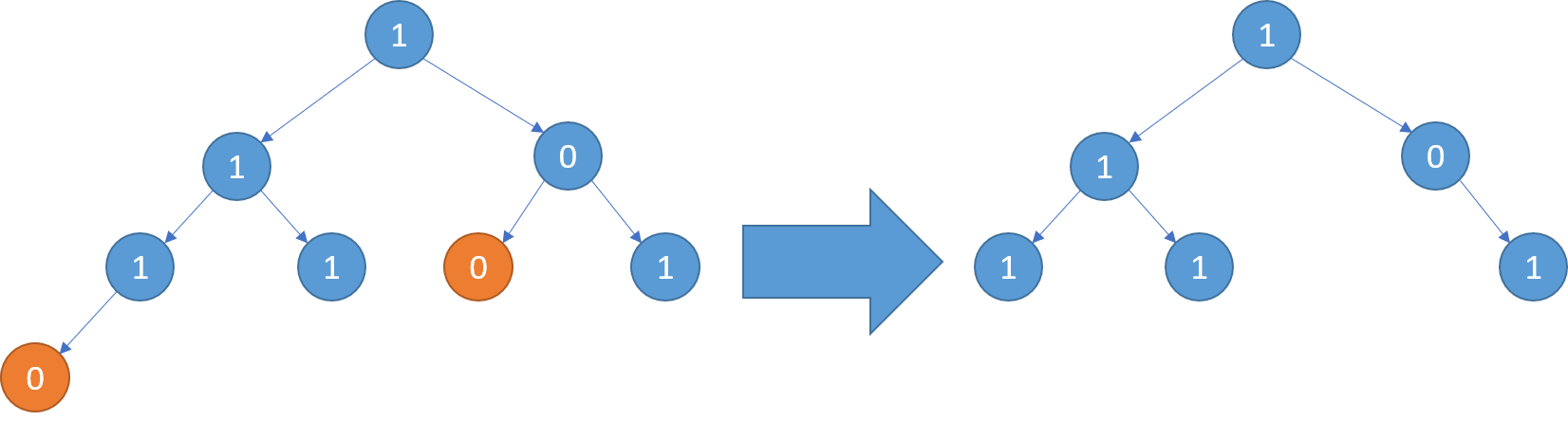# LeetCode: 814. Binary Tree Pruning

## 题目¶

We are given the head node root of a binary tree, where additionally every node's value is either a 0 or a 1.

Return the same tree where every subtree (of the given tree) not containing a 1 has been removed.

(Recall that the subtree of a node X is X, plus every node that is a descendant of X.)

Example 1:

```Input: [1,null,0,0,1]
Output: [1,null,0,null,1]
```

Explanation:

Only the red nodes satisfy the property "every subtree not containing a 1". The diagram on the right represents the answer.Example 2:

```Input: [1,0,1,0,0,0,1]
Output: [1,null,1,null,1]
```Example 3:

```Input: [1,1,0,1,1,0,1,0]
Output: [1,1,0,1,1,null,1]
```Note:

• The binary tree will have at most 200 nodes.
• The value of each node will only be 0 or 1.

## 解法¶

```# Definition for a binary tree node.
# class TreeNode(object):
#     def __init__(self, val=0, left=None, right=None):
#         self.val = val
#         self.left = left
#         self.right = right
class Solution(object):
def pruneTree(self, root):
if root is None:
return

# 将不满足条件的节点置为 None
if not (root.val == 1 or
(root.left is not None and root.left.val == 1) or
(root.right is not None and root.right.val == 1)):
return

root.left = self.pruneTree(root.left)
root.right = self.pruneTree(root.right)

return root
```